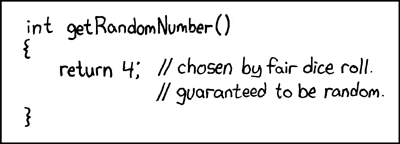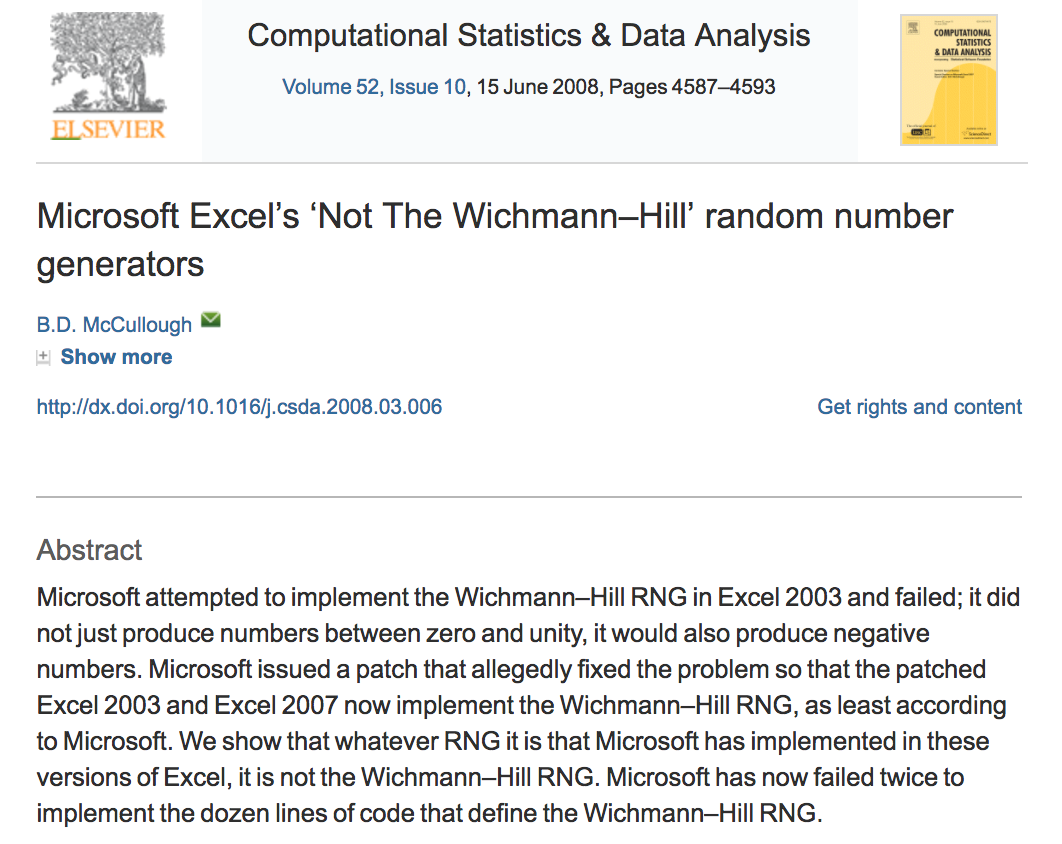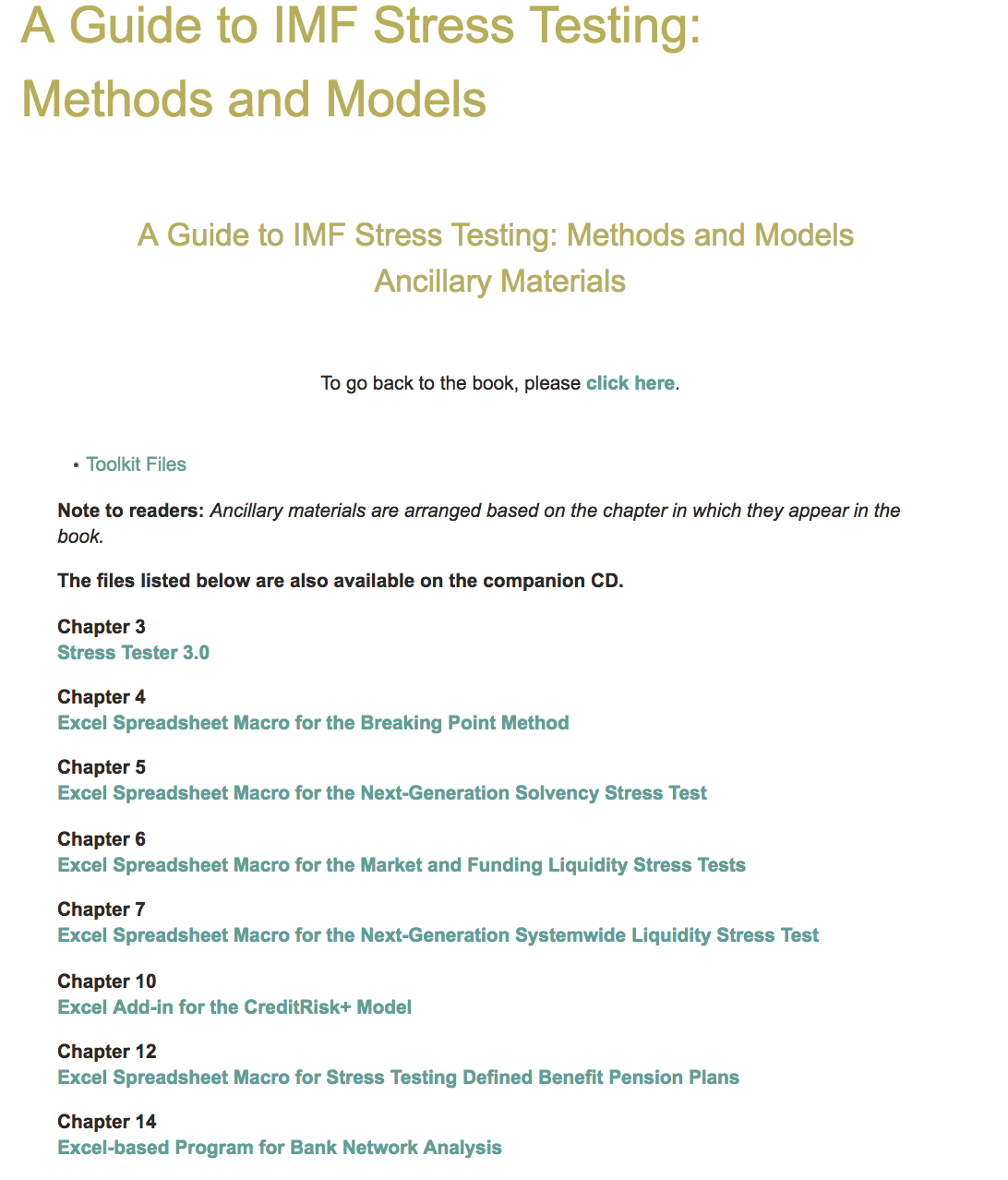# With Great Power Comes Great Responsibility: Multivariate Permutation Tests and their Numerical Implementation¶

## International Society of Nonparametric Statistics (ISNPS)¶

### 11–15 June 2018

1. Generic permutation tests
2. Estimating $P$-values by simulation–in theory
3. Numerical implementation: generating random transformations
4. PRNGs
5. Pseudo-random integers
6. Pseudo-random permutations and sampling

## Generic permutation test¶

• Data $X \sim F_X$ takes values in $\mathcal{X}$.
• $\mathcal{G}$ is a group of transformations from $\mathcal{X}$ to $\mathcal{X}$
• Probability measure $\mu$ on $\mathcal{G}$ that is left and right invariant: If $G \subset \mathcal{G}$ is measurable, then $\forall g \in \mathcal{G}$, $gG$ and $Gg$ are measurable, and $\mu(G) = \mu(gG) = \mu(Gg)$.
• Under $H_0$, $\forall g \in \mathcal{G}$, $X \sim gX$
• $T: \mathcal{X} \rightarrow \mathbb{R}$ is a test statistic on $\mathcal{X}$; large values considered evidence against $H_0$
• Observe $X = x$.
• $p = \mu \{g \in \mathcal{G}: T(gx) \ge Tx \}$ is $P$-value of $H_0$, conditional on $X \in \mathcal G x$.

## Estimating $P$-values by simulation¶

• Generate $\{G_j \}$ IID $\mu$
• $1_{T(G_jx) \ge Tx} \sim \mbox{Bernoulli}(P)$
• tests about $P$ (incl. sequential tests); upper confidence bounds

## Generating $G \sim \mu$ in practice¶

The difference between theory and practice is smaller in theory than it is in practice.
—unknown
In theory, there's no difference between theory and practice, but in practice, there is.
—Jan L.A. van de Snepscheut

### PRNGs¶## Properties of PRNGs¶

• dimension of output: commonly 32 bits, but some have more
• number of states
• some PRNGs have state = output
• better generators generally have output = f(state)
dim(state) $>>$ dim(output)
• period
• maximum over initial states of the number of states visited before repeating
• period ≤ #states ($<<$ for some PRNGs/seeds)
• sensitivity to initial state; burn-in
• many PRNGs don't do well if the seed has too many zeros
• some require many iterations before output behaves well

## Some PRNGs¶

Anyone who considers arithmetical methods of producing random digits is, of course, in a state of sin.
—John von Neumann
• Linear congruential (LCG) $X_{n+1} = (aX_n +c)\mod m.$ Period $\le m$.

• Lagged Fibonacci, KISS, xorshift family, PCG, ...

• Wichmann-Hill Sum of 3 LCGs.

def WH(s1, s2, s3):
s1 = (171 * s1) % 30269
s2 = (172 * s2) % 30307
s3 = (170 * s3) % 30323
r = (s1/30269 + s2/30307 + s3/30323) %  1
return [r, s1, s2, s3]McCullough, B.D., 2008. Microsoft Excel's 'Not The Wichmann–Hill' random number generators Computational Statistics & Data Analysis, 52, 4587–4593 doi:10.1016/j.csda.2008.03.006### Mersenne Twister (MT) Matsumoto & Nishimura (1997)¶

• state space 19937 bits

• period $2^{19937}-1$, a Mersenne Prime

• can have slow "burn in," especially for seeds with many zeros

• output for close seeds can be close

• perfectly predictable from 624 successive outputs; problems discovered in 2007

• default in: GNU Octave, Maple, MATLAB, Mathematica, Python, R, Stata, many more

## Cryptographic hash functions¶

1. fixed-length "digest" from arbitrarily long "message": $H:\{0, 1\}^* \rightarrow \{0, 1\}^L$.
2. inexpensive to compute
3. non-invertible ("one-way," hard to find pre-image of any hash except by exhaustive enumeration)
4. collision-resistant (hard to find $M_1 \ne M_2$ such that $H(M_1) = H(M_2)$)
5. small change to input produces big change to output ("unpredictable," input and output effectively independent)
6. equidistributed: bits of the hash are essentially random

As if $H(M)$ is random $L$-bit string is assigned to $M$ in a way that's essentially unique.

#### Simple, hash-based PRNG¶

Generate a random string $S$ of reasonable length, e.g., 20 digits.

$$X_i = {\mbox{Hash}}(S,i),$$

interpreted as a (long) hexadecimal number.

Unbounded state space!

### Generating a $U\{1, \ldots, m\}$ variable¶

Textbook: take $X \sim U[0,1)$; define $Y \equiv 1 + \lfloor mX \rfloor$.

In practice, $X$ from a PRNG is not really $U[0,1)$: derived by normalizing a PRN that's (supposed to be) uniform on $w$-bit integers.

• If $m > 2^w$, at least $m-2^w$ values will have probability 0 instead of probability $1/m$.

• Unless $m$ is a power of 2, the distribution of $Y$ isn't uniform on $\{1, \ldots, m\}$.

• For $m < 2^w$, the ratio of the largest to smallest selection probability is, to first order, $1+ m 2^{-w}$. (Knuth v2 3.4.1.A.)

• For $m = 10^9$ and $w=32$, $1 + m 2^{-w} \approx 1.233$.

• If $w=32$, then for $m>2^{32}=4.24e9$, some values will have probability 0.

• Until relatively recently, R did not support 64-bit integers.

#### Better method¶

Generate $\log_2(m-1)$ pseudo-random bits; discard out-of-range numbers.

numpy.random.randint() does this, but numpy.random.choice() doesn't

In R,

sample(1:m, k, replace=FALSE)



uses variant of the faulty 1 + floor(m*X) approach.

## Pigeonhole principle¶

If you put $N>n$ pigeons in $n$ pigeonholes, then at least one pigeonhole must contain more than one pigeon.

## Corollary¶

At most $n$ pigeons can be put in $n$ pigeonholes if at most one pigeon is put in each hole.

## Permutations¶

Stirling bounds $e n^{n+1/2} e^{-n} \ge n! \ge \sqrt{2 \pi} n^{n+1/2} e^{-n}.$

## Sampling without replacement¶

#### Entropy bounds.¶

$\frac{2^{nH(k/n)}}{n+1} \le {n \choose k} \le 2^{nH(k/n)},$ where $H(q) \equiv -q \log_2(q) - (1-q) \log_2 (1-q)$.

#### Stirling bounds¶

For $\ell \ge 1$ and $m \ge 2$, ${ {\ell m } \choose { \ell }} \ge \frac{m^{m(\ell-1)+1}}{\sqrt{\ell} (m-1)^{(m-1)(\ell-1)}}.$

## Sampling with replacement¶

$n^k$ possible samples of size $k$ from $n$ items

Expression full scientific notation
$2^{32}$ 4,294,967,296 4.29e9
$2^{64}$ 18,446,744,073,709,551,616 1.84e19
$2^{128}$ 3.40e38
$2^{32 \times 624}$ 9.27e6010
$13!$ 6,227,020,800 6.23e9
$21!$ 51,090,942,171,709,440,000 5.11e19
$35!$ 1.03e40
$2084!$ 3.73e6013
${50 \choose 10}$ 10,272,278,170 1.03e10
${100 \choose 10}$ 17,310,309,456,440 1.73e13
${500 \choose 10}$ 2.4581e20
$\frac{2^{32}}{{50 \choose 10}}$ 0.418
$\frac{2^{64}}{{500 \choose 10}}$ 0.075
$\frac{2^{32}}{7000!}$ $<$ 1e-54,958
$\frac{2}{52!}$ 2.48e-68

## $L_1$ bounds¶

Suppose ${\mathbb P}_0$ and ${\mathbb P}_1$ are probability distributions on a common measurable space.

If there is some set $S$ for which ${\mathbb P}_0 = \epsilon$ and ${\mathbb P}_1(S) = 0$, then $\|{\mathbb P}_0 - {\mathbb P}_1 \|_1 \ge 2 \epsilon$.

Thus there is a function $f$ with $|f| \le 1$ such that

$${\mathbb E}_{{\mathbb P}_0}f - {\mathbb E}_{{\mathbb P}_1}f \ge 2 \epsilon.$$

• If PRNG has $n$ states and want to generate $N>n$ equally likely outcomes, at least $N-n$ outcomes will have probability zero instead of $1/N$.
• $\| \mbox{true} - \mbox{desired} \|_1 \ge 2 \times \frac{N-n}{N}$

### Random sampling¶

Given a good source of randomness, many ways to draw a simple random sample.

One basic approach: shuffle then first $k$.

Many ways to generate pseudo-random permutation.

### Algorithm PIKK (permute indices and keep $k$)¶

For instance, if we had a way to generate independent, identically distributed (iid) $U[0,1]$ random numbers, we could do it as follows:

Algorithm PIKK

• assign iid $U[0,1]$ numbers to the $n$ elements of the population
• sort on that number (break ties randomly)
• the sample consists of first $k$ items in sorted list
• amounts to generating a random permutation of the population, then taking first $k$.
• if the numbers really are iid, every permutation is equally likely, and first $k$ are an SRS
• requires $n$ random numbers (and sorting)

More efficient:

Algorithm Fisher-Yates-Knuth-Durstenfeld shuffle (backwards version)

for i=1, ..., n-1:
J <- random integer uniformly distributed on {i, ..., n}
(a[J], a[i]) <- (a[i], a[J])


• need independent uniform integers on various ranges
• no sorting

There's a version suitable for streaming, i.e., generating a random permutation of a list that has an (initially) unknown number $n$ of elements:

Algorithm Fisher-Yates-Knuth-Durstenfeld shuffle (streaming version)

i <- 0
a = []
while there are records left:
i <- i+1
J <- random integer uniformly distributed on {1, ..., i}
if J < i:
a[i] <- a[J]
a[J] <- next record
else:
a[i] <- next record

## A good way to get a bad shuffle¶

Sort using a "random" comparison function, e.g.,

def RandomSort(a,b):
return (0.5 - np.random.random())



But this fails the basic properties of an ordering, e.g., transitivity, reflexiveness, etc. Doesn't produce random permutation. Output also depends on sorting algorithm.

The right way, the wrong way, and the Microsoft way.

Notoriously used by Microsoft to offer a selection of browsers in the EU. Resulted in IE being 5th of 5 about half the time in IE and about 26% of the time in Chrome, but only 6% of the time in Safari (4th about 40% of the time).

### Cormen et al. (2009) Algorithm Random_Sample¶

• recursive algorithm
• requires only $k$ random numbers (integers)
• no sorting
In :
def Random_Sample(n, k, gen=np.random):  # from Cormen et al. 2009
if k==0:
return set()
else:
S = Random_Sample(n-1, k-1)
i = gen.randint(1,n+1)
if i in S:
S = S.union([n])
else:
S = S.union([i])
return S

Random_Sample(100,5)

Out:
{11, 15, 35, 43, 48}

## Reservoir algorithms¶

The previous algorithms require $n$ to be known. There are reservoir algorithms that do not. Moreover, the algorithms are suitable for streaming (aka online) use: items are examined sequentially and either enter into the reservoir, or, if not, are never revisited.

## Algorithm R, Waterman (per Knuth, 1997)¶

• Put first $k$ items into the reservoir

• when item $k+j$ is examined, either skip it (with probability $j/(k+j)$) or swap for a uniformly selected item in the reservoir (with probability $k/(k+j)$)

• naive version requires at most $n-k$ pseudo-random numbers

• closely related to FYKD shuffle

## Algorithm Z, Vitter (1985)¶

Much more efficient than Algorithm R, using random skips. Essentially linear in $k$.

Note: Vitter proposes using the (inaccurate) $J = \lfloor mU \rfloor$ to generate a random integer between $0$ and $m$ in both algorithm R and algorithm Z. Pervasive!

## PRNGs in Common packages¶

Package/Lang default other SRS algorithm
SAS 9.2 MT 32-bit LCG Floyd's ordered hash or Fan et al. 1962
SPSS 20.0 32-bit LCG MT1997ar trunc + rand indices
SPSS ≤ 12.0 32-bit LCG
STATA 13 KISS 32 PIKK
STATA 14 MT PIKK
R MT trunc + rand indices
python MT mask + rand indices
MATLAB MT trunc + PIKK
StatXact MT

Key. MT = Mersenne Twister. LCG = linear congruential generator. PIKK = assign a number to each of the $n$ items and sort. The KISS generator combines 4 generators of three types: two multiply-with-carry generators, the 3-shift register SHR3 and the congruential generator CONG.

STATA 10 before April 2011: 95.1% of the $2^{31}$ possible seed values resulted in the first and second draws from rnormal() having the same sign.

## Is MT adequate for statistics?¶

• Know from pigeonhole argument that $L_1$ distance between true and desired is big for modest sampling & permutation problems.

• Know from equidistribution of MT that large ensemble frequencies will be right, but expect dependence issues

• Looking for problems that occur across seeds, large enough to be visible in $O(10^5)$ replications

• Examined simple random sample frequencies, derangements, partial derangements, Spearman correlation, etc.

• Must be problems, but where are they?

## Best Practices¶

• Use a source of real randomness to set the seed with a substantial amount of entropy, e.g., 20 rolls of 10-sided dice.
• Record the seed so your analysis is reproducible.
• Use a PRNG at least as good as the Mersenne Twister, and preferably a cryptographically secure PRNG. Consider the PCG family.
• Avoid standard linear congruential generators and the Wichmann-Hill generator.
• Use open-source software, and record the version of the software.
• Use a sampling algorithm that does not "waste randomness." Avoid permuting the entire population.
• Be aware of discretization issues in the sampling algorithm; many methods assume the PRNG produces $U[0,1]$ or $U[0,1)$ random numbers, rather than (an approximation to) numbers that are uniform on $w$-bit binary integers.
• Consider the size of the problem: are your PRNG and sampling algorithm adequate?
• Avoid "tests of representativeness" and procedures that reject some samples. They alter the distribution of the sample.

## Recommendations¶

• Replace the standard PRNGs in R and Python with PRNGs with unbounded state spaces, and cryptographic or near-cryptographic quality

• Consider using AES in counter mode, since Intel chips have hardware support for AES
• Replace floor(1+nU) in R's sample() with bit-mask algorithm

1. Argyros, G. and A. Kiayias, 2012. PRNG: Pwning Random Number Generators. https://media.blackhat.com/bh-us-12/Briefings/Argyros/BH_US_12_Argyros_PRNG_WP.pdf
2. Bernstein, D.J., T. Lange, and R. Niederhagen, 2016. Dual EC: A Standardized Back Door, in The New Codebreakers, Essays Dedicated to David Kahn on the Occasion of his 85th Birthday, Ryan, P.Y.A., D. Naccache, and J-J Quisquater, eds., Springer, Berlin.
3. Cormen, T.H., C.E. Leiserson, R.L. Rivest and C. Stein, 2009. Introduction to Algorithms, 3rd edition, MIT Press.
4. Fishman, G.S., and L.R. Moore, 1981. In Search of Correlation in Multiplicative Congruential Generators with Modulus 2**31-1, Computer Science and Statistics: Proceedings of the 13 Symposium on the Interface, William F. Eddy, ed., Springer Verlag, New York.
5. Knuth, D., 1997 The Art of Computer Programming, V.II: Seminumerical methods, 3rd edition, Addison-Wesley, Boston.
6. L'Ecuyer, P. and R. Simard, 2007. TestU01: A C Library for Empirical Testing of Random Number Generators, ACM Trans. Math. Softw., 33, http://doi.acm.org/10.1145/1268776.1268777
7. Marsaglia, G., 1968. Random Numbers Fall Mainly in the Planes, PNAS, 61, 25–28.
8. Marsaglia, G., 2003. Xorshift RNGs. Journal of Statistical Software, 8, 1–6.
9. Matsumoto, M., and T. Nishimura, 1998. 8). Mersenne twister: a 623-dimensionally equidistributed uniform pseudo-random number generator, ACM Transactions on Modeling and Computer Simulation, 8, 3–30. doi:10.1145/272991.272995
10. McCullough, B.D., 2008. Microsoft's 'Not the Wichmann-Hill' random number generator. Computational Statistics and Data Analysis, 52 (10), 4587–4593. http://dx.doi.org/10.1016/j.csda.2008.03.006
11. NIST Computer Security Division, Random Number Generation http://csrc.nist.gov/groups/ST/toolkit/rng/
12. O'Neill, M.E., 2015. PCG: A Family of Simple Fast Space-Efficient Statistically Good Algorithms for Random Number Generation, submitted to ACM Transactions on Mathematical Software. http://www.pcg-random.org/pdf/toms-oneill-pcg-family-v1.02.pdf
13. http://www.pcg-random.org/
14. Shannon, C.E., 1948. A Mathematical Theory of Communication, Bell System Technical Journal, 27, 379–423, 623–656.
15. Vitter, J.S., 1985. Random Sampling with a Reservoir, ACM Transactions on Mathematical Software, 11, 37–57.
16. Wikipedia articles, including https://en.wikipedia.org/wiki/Mersenne_Twister, https://en.wikipedia.org/wiki/Linear_congruential_generator, https://en.wikipedia.org/wiki/Comparison_of_hardware_random_number_generators, https://en.wikipedia.org/wiki/Pseudorandom_number_generator, https://en.wikipedia.org/wiki/List_of_random_number_generators, https://en.wikipedia.org/wiki/Random_number_generator_attack